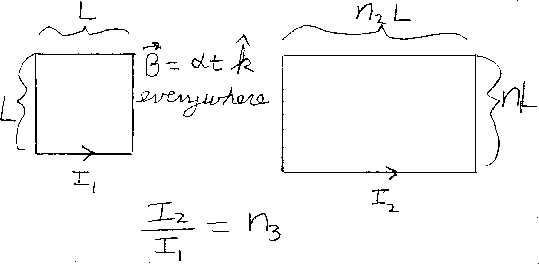Problem C8: The two loops of wire lie in the same plane. The wire on the left is a square with sides of length L. The wire on the right is a rectangle with sides n1L by n2L. The wires in each loop have the same cross section and electrical resistivity. There is a magnetic field throughout the region that is steadily increasing in time: B=α t k, where k points out of the page. What is the ratio of the current induced in the right wire, I2, divided by the current induced in the left wire, I1? If I2/I1 = n3, then what is n3? Neglect any mutual inductance between the wires and self inductance of the wire loops.n1 = n2 = Input n3:
If you are currently in my class, you can record your grade by entering your name and student ID number (without the leading zeros) below and clicking on "record grade".
 First Name = Last Name = ID = Problem: